index: click on a letter A B C D E F G H I J K L M N O P Q R S T U V W X Y Z A to Z index index: subject areas numbers & symbols sets, logic, proofs geometry algebra trigonometry advanced algebra & pre-calculus calculus advanced topics probability & statistics real world applications multimedia entrieswww.mathwords.com about mathwords website feedback

Square Root Rules

Algebra rules for square roots are listed below. Square root rules are a subset of nth root rules and exponent rules.

 Definitions 1.if both b ≥ 0 and b2 = a. 2.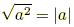Examplesbecause 32 = 9.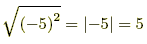3. If a ≥ 0 then.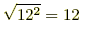Distributing (a ≥ 0 and b ≥ 0) 1.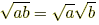2.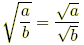(b ≠ 0) 3.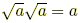Examples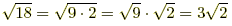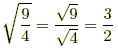4.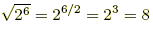Rationalizing the Denominator (a > 0, b > 0, c > 0) Examples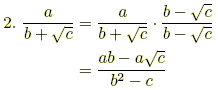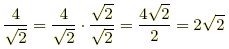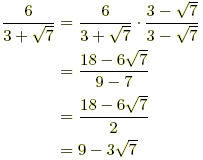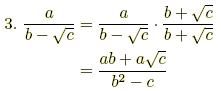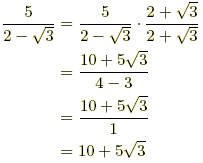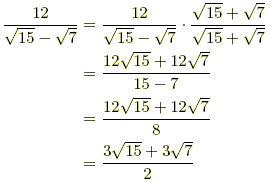Careful!! 1.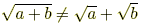2.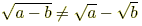3.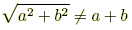Examples